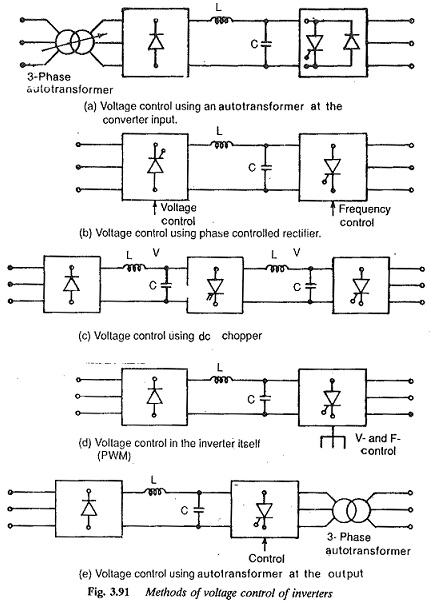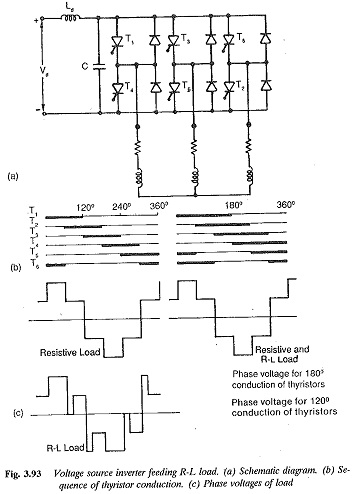# Three Phase Inverter

## Three Phase Inverter:

The variable frequency required for the speed control of three phase ac motors is obtained from a Three Phase Inverter. To avoid magnetic saturation and to obtain constant flux conditions in the machine, the voltage fed to the motor must also be varied. Therefore an inverter feeding a three phase motor must be capable of providing a variable voltage, variable frequency supply. The required voltage control can be obtained either external to the inverter or within it (Fig. 3.91). In the former, the input voltage to the inverter is variable, whereas in the latter it is constant and the required variable voltage at the output terminals is obtained by controlling the inverter.The dc voltage to the inverter is normally obtained by rectifying a 50 Hz supply using a bridge rectifier. The rectifier and inverter are interconnected by means of energy storing elements. These provide a kind of decoupling between the rectifier and the inverter. Such converters are called dc link converters. They provide a variable voltage, variable frequency supply from constant voltage, constant frequency mains, and are two stage conversion de­vices. The inductance in the dc link smoothens the current while the capacitor maintains the voltage constant.

Since the voltage is a controlled quantity, these are called voltage source inverters. When the voltage control is done external to the inverter, the line side rectifier must be a phase controlled one. By varying the firing angle the output voltage of the rectifier, and hence the input voltage to the inverter, can be varied. These are called square wave or variable voltage inverters. If the voltage control is available in the inverter itself, the input voltage of the Three Phase Inverter is constant and a simple diode rectifier suffices on the line side.The Three Phase Inverter uses PWM for voltage control and hence is called a PWM inverter or constant voltage inverter (Fig. 3.93). In Three Phase Inverter the voltage is maintained constant at a controlled value, irrespective of the load events. The capacitance across the inverter maintains the constant voltage.